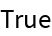#Function Repository Resource:

# ComplexRoots

Get the full list of complex nth roots of a number

Contributed by: Wolfram|AIpha Math Team
 ResourceFunction["ComplexRoots"][x,n] gives a list of the nth roots of a complex number x.

## Examples

### Basic Examples

Find the three third roots of unity:

 In:=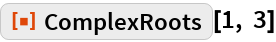Out=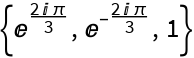Find the sixth roots of 2:

 In:=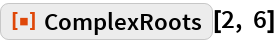Out=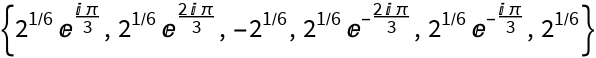Find the fifth roots of -1:

 In:=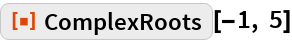Out=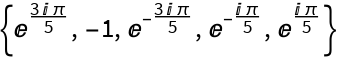### Properties and Relations

Exact values are returned for exact input:

 In:=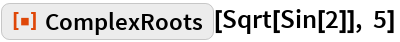Out=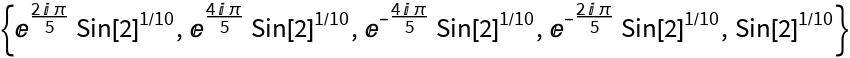Use ResourceFunction["ComplexRootQ"] to confirm the output of ComplexRoots:

 In:=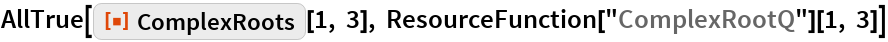Out=